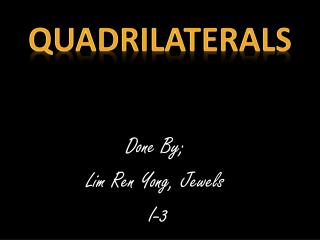DownloadDownload PresentationDone By; Lim Ren Yong, Jewels I-3

# Done By; Lim Ren Yong, Jewels I-3

Download Presentation## Done By; Lim Ren Yong, Jewels I-3

- - - - - - - - - - - - - - - - - - - - - - - - - - - E N D - - - - - - - - - - - - - - - - - - - - - - - - - - -
##### Presentation Transcript

1. Quadrilaterals Done By; Lim Ren Yong, Jewels I-3

2. Quadrilateral just means "four sides“(quad means four, lateral means side). Any four-sided shape is a Quadrilateral. But the sides have to be straight, and it has to be 2-dimensional. Quadrilaterals

3. ~Properties~ • Four sides (or edges) • Four vertex (or corners). • The interior angles add up to 360 degrees • All angles should add up to 360 degrees

4. Types of Quadrilaterals There are special types of quadrilateral: -Square -Kite -Parallelogram -Rectangle -Rhombus -Trapezium • Some types are also included in the definition of other types. For example a square, rhombusand rectangle are also parallelograms

5. Rectangles -The square represents the right angle -The lines shows the equal sides of the rectangle A rectangle is a four-sided shape where every angle is a right angle(90°). Also opposite sides areparalleland of equal length.

6. -Rhombus- • ARhombus is a four-sided shape where all sides have equal length. • Also opposite sides are parallel and opposite angles are equal. • Another interesting thing is that the diagonals (dashed lines in second figure) of a rhombus bisect each other at right angles.

7. Square -The small square represents the right angle -The lines shows the equal sides of the rectangle • A square has equal sides and every angle is a right angle (90°) • Also opposite sides are parallel. • A square also fits the definition of a rectangle (all angles are 90°), and a rhombus (all sides are equal length).

8. Parallelogram NOTE: Squares, Rectangles and Rhombuses are all Parallelograms! • Opposite sides are parallel and equal in length, and opposite angles are equal (angles "a" are the same, and angles "b" are the same) Example; A parallelogram with: -All sides equal -Angles "a" and "b" as right angles is a square

9. -It is called an Isosceles trapezium if the sides that aren't parallel are equal in length and both angles coming from a parallel side are equal, as shown. A trapezium has one pair of opposite sides parallel. TRAPEZIUM

10. The kite has two pairs of sides. Each pair is made up of sides that are equal in length. The angles are equal where the pairs meet. Diagonals (the dashed lines) meet at a right angle, and one of the diagonal bisects (cuts equally in half) the other. The small square represents the right angle The lines shows the equal sides of the kite Kite

11. Thank You! For watching the PowerPoint slides!